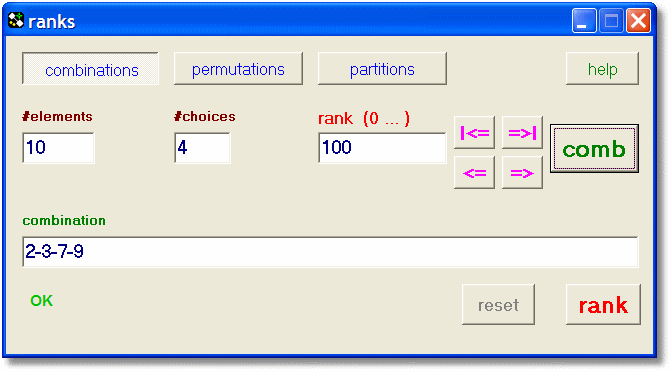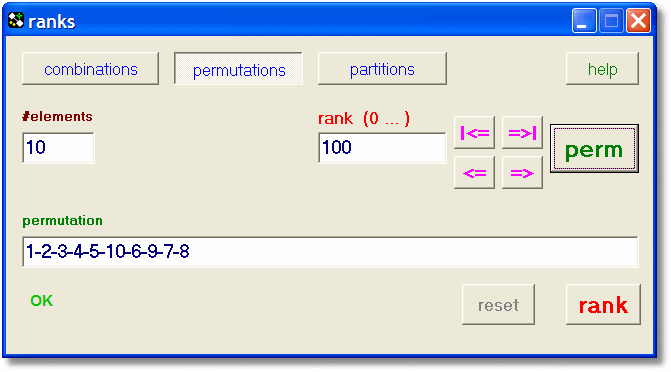downloadRANKS RanksIntroduction

Ranks is a program that assigns a sequential number (rank) to a combination, permutation or partition.

Given a rank, the combination permutation or partition may be generated.
Given a combination permutation or partition, the rank may be calculated.

Ranks is freeware and may be copied without restriction.

Installation

Ranks ships as a single .exe file.
It contains In-Line help information.
The system is Windows 95 and up.
Minimum screen resolution is 600*800.

There is no installation procedure, just copy to a map of choice.
The Windows Registry is not changed.
The size is 268kB.

Combinations

A combination is a selection of elements from a set, where each element may be choosen once
and the sequence of selection is unimportant.
In this program (ranks) the elements are always the natural numbers 1,2,3,4,......
The maximum number of elements is 50.

The ranking of combinations may be usefull in the analyses of lotto games or any case where
combinations have to be generated in a systematical way.

The number of possible combinations of k elements from a set of n is written as
C(n,k) = n!/(k!*(n-k)!)
Below, all combinations of 2 elements from a set of 4 are listed together with the rank of that combination.

 rank combination 0 1-2 1 1-3 2 1-4 3 2-3 4 2-4 5 3-4
There are 6 possible combinations, the ranks are 0 to 5.4 elements of 10: rank 100

Permutations

A permutation is a sequence of elements.
Elements are always named 1,2,3,4,....
The maximum number of elements is 12.

n elements have n! permutations, where n! = n.(n-1).(n-2)........(3).(2).(1)

Ranking a permutation is usefull when sequences have to be generated in a systematical way,
as is the case in logigram puzzle solving.

Below are listed all permutations of a set of 4 elements
 rank permutation 0 1-2-3-4 1 1-2-4-3 2 1-3-2-4 3 1-3-4-2 4 1-4-2-3 5 1-4-3-2 6 2-1-3-4 7 2-1-4-3 8 2-3-1-4 9 2-3-4-1 10 2-4-1-3 11 2-4-3-1 12 3-1-2-4 13 3-1-4-2 14 3-2-1-4 15 3-2-4-1 16 3-4-1-2 17 3-4-2-1 18 4-1-2-3 19 4-1-3-2 20 4-2-1-3 21 4-2-3-1 22 4-3-1-2 23 4-3-2-1
So, there are 24 permutations, ranked 0 to 23.permutation of 10 elements: rank 100

Partitions

A partition of a number n is a list of numbers which have a sum of n.
The sequence of the numbers is not important so they are shown sorted.

The maximal number is 50.

Ranking a partition may be usefull in cases where partitions have to be generated in a
systematical way, which is needed in the analyses of certain Nim games.

Below are listed all partitions of the number 6, together with the ranks.
 rank partition 0 6 1 5-1 2 4-2 3 4-1-1 4 3-3 5 3-2-1 6 3-1-1-1 7 2-2-2 8 2-2-1-1 9 2-1-1-1-1 10 1-1-1-1-1-1
Note, that the partitions are sorted.
Number 6 has 11 partitions, ranked 0 to 10.number 10: partition number 30

How it works

Table below lists some type definitions and variables.

 ```type Taction = (acComb,acPerm,acPart); Taa = array[1..50] of byte; var action : Taction = acComb; elements : Taa; partList : Taa; //make next partition until = elements pmax : byte; //scratch for partitions faculties : array[0..12] of longInt; number : byte; choices : byte; rank : longInt; maximum : boolean = false; ```
Warning: an error has been reported in some Delphi versions were the variable "action" is used already.
Rename "action" to avoid this conflict.

Note: faculties[n] is set to n! on creation of the form.
Note: elements[ ] holds the combination, permutation or partition.

number,choice and rank are the numeric values of edit components on the form.

Calculating the combination from a rank
 ```function C(n,k : byte) : longInt; //calculate combinations k of n var i : byte; tt,nn : double; begin if (k = 0) or (k = n) then begin result := 1; exit; end; //-- if 2*k > n then k := n - k; tt := 1; nn := 1; try for i := 0 to k-1 do begin tt := tt * (n-i); nn := nn * (k-i); end; result := trunc(tt/nn); except result := 0; end; end; procedure newComb; var r,comb : longInt; i,n,k : byte; elList : array[1..50] of byte; endrepeat : boolean; begin if Checkelements then exit; if checkChoices then exit; if CheckRank then exit; //---- r := rank; k := 1; if rank = C(number,choices)-1 then maximum := true else maximum := false; clearelements(elements); for i := 1 to 50 do ElList[i] := i; //---- for n := 1 to choices do begin endrepeat := false; repeat comb := C(number-k,choices-n); if r >= comb then begin inc(k); r := r - comb; end else endrepeat := true; ; until endrepeat; elements[n] := ElList[k]; inc(k); end;//for n //--- ShowElements(choices); end; ```

Calculating the rank of a combination

 ```procedure newCombRank; //calulate rank from combination var i,j,min : byte; begin if checkelements then exit; if getCombination then exit; if checkchoices then exit; //-- for i := 1 to choices do if (elements[i] = 0) or (elements[i] > number) then begin post(16); exit; end; //-- for i := 1 to choices-1 do if elements[i] = elements[i+1] then begin post(17); exit; end; //-- min := 1; rank := 0; for i := 1 to choices do begin for j := min to elements[i]-1 do rank := rank + C(number-j,choices-i); min := elements[i]+1; end; //-- form1.edit4.text := inttostr(rank); post(6); end; ```

Calculating the permutatation of a rank

 ```procedure newPerm; //make permutation from number, rank var i,k,x : byte; r : longInt; list : array[1..12] of byte; begin if CheckElements then exit; if CheckRank then exit; //----- r := rank; if rank = faculties[number]-1 then maximum := true else maximum := false; clearElements(elements); for i := 1 to 12 do list[i] := i; //-- for i := 1 to number do begin x := r div faculties[number-i] + 1; r := r mod faculties[number-i]; elements[i] := list[x]; for k := x to 11 do list[k] := list[k+1]; end; //-- ShowElements(number); end; ```

Calculating the rank of a permutation

 ```procedure newPermRank; var i,j : byte; list : array[1..12] of byte; begin if checkelements then exit; if GetPermutation then exit; //-- for i := 1 to 12 do list[i] := i; rank := 0; for i := 1 to number do begin j := 1; while elements[i] <> list[j] do inc(j); rank := rank + (j-1)*faculties[number-i]; for j := j to 11 do list[j] := list[j+1]; end; form1.edit4.text := inttostr(rank); post(6); end; ```

Calculating the partition of a rank

 ```procedure newPart; var n : longInt; max,min,acc : byte; s : string; begin if CheckElements then exit; if CheckRank then exit; //-- clearElements(elements); maximum := false; elements := number; max := 1; for n := 1 to rank do begin acc := 0; while (max > 0) and (elements[max] = 1) do begin dec(max); inc(acc); end; if elements = 1 then begin maximum := true; rank := n-1; form1.edit4.text := inttostr(rank); showElements(number); exit; end; //----- dec(elements[max]); inc(acc); min := elements[max]; while acc > 0 do begin inc(max); if acc >= min then begin elements[max] := min; acc := acc - min; end else begin elements[max] := acc; acc := 0; end; end;//while end;//for ShowElements(max); end; ```

Calculating the rank of a partition

 ```procedure NextPartition; //generate next partition in partlist var n : longInt; min,acc : byte; s : string; begin acc := 0; while (pmax > 0) and (partlist[pmax] = 1) do begin dec(pmax); inc(acc); end; dec(partlist[pmax]); inc(acc); min := partlist[pmax]; while acc > 0 do begin inc(pmax); if acc >= min then begin partlist[pmax] := min; acc := acc - min; end else begin partlist[pmax] := acc; acc := 0; end; end;//while end; procedure newPartRank; var i,count,sum : byte; begin if GetPartition(count) then exit; if checkelements then exit; for i := 2 to 50 do partlist[i] := 0; partlist := number; pmax := 1; rank := 0; //-- repeat sum := 0; for i := 1 to count do if partlist[i] = elements[i] then inc(sum) else break; if sum <> count then begin inc(rank); NextPartition; end; until sum = count; form1.Edit4.text := inttostr(rank); post(6); end; ```
Note: I do not know a direct way to generate a partition from a rank.
Partitions are generated sequentially, starting from (rank) 0.

The Delphi-7 project

Interested in the complete source code of the ranks project? Click [ here ] to download.